# V - slope

The slope of the line whose equation is -3x -9 = 0 is

Result

k = (Correct answer is: ∞)### Step-by-step explanation:

$k=\frac{\mathrm{\Delta }y}{\mathrm{\Delta }x}=\frac{\mathrm{\Delta }y}{0}=\mathrm{\infty }$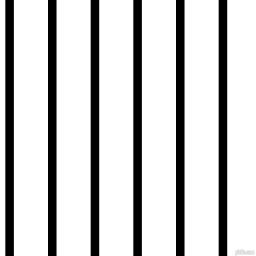Did you find an error or inaccuracy? Feel free to write us. Thank you!

Showing 1 comment:Aesthetic_princess377
Which line has a slope of - 1/2

line a
line b
line c
line dTips to related online calculators
Line slope calculator is helpful for basic calculations in analytic geometry. The coordinates of two points in the plane calculate slope, normal and parametric line equation(s), slope, directional angle, direction vector, the length of the segment, intersections of the coordinate axes, etc.
Check out our ratio calculator.

## Related math problems and questions:

• SlopeWhat is the slope of the line defined by the equation -2x +3y = -1 ?
• CoordinatesDetermine the coordinates of the vertices and the content of the parallelogram, the two sides of which lie on the lines 8x + 3y + 1 = 0, 2x + y-1 = 0 and the diagonal on the line 3x + 2y + 3 = 0
• General line equationsIn all examples, write the GENERAL EQUATION OF a line that is given in some way. A) the line is given parametrically: x = - 4 + 2p, y = 2 - 3p B) the line is given by the slope form: y = 3x - 1 C) the line is given by two points: A [3; -3], B [-5; 2] D) t
• Roots count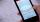Substitute the numbers/0,1,2,3/into the equation as x: (x - 1) (x - 3) (x + 1) = 0 Which of them is its solution? Is there another number that solves this equation?
• What is 10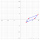What is this The area of a parallelogram that has vertices with the coordinates (0, 0), (4, 0),(5, 3), and (1, 3)?
• Slope formFind the equation of a line given the point X(8, 1) and slope -2.8. Arrange your answer in the form y = ax + b, where a, b are the constants.
• Using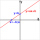Using the point-slope equation, find the equation containing (-7, 3) and slope m = -4
• Line segmentFor the line segment whose endpoints are L[-1, 13] and M[18, 2], find the x and y value for the point located 4 over 7 the distance from L to M.
• Slope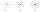Calculate the slope of a line that intersects points (-84,41) and (-76,-32).
• LineLine p passing through A[-10, 6] and has direction vector v=(3, 2). Is point B[7, 30] on the line p?
• Slope of the pool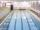Calculate slope (rise:run) of the bottom of the swimming pool long 30 m. Water depth at beginning of the pool is 1.13 m (for children) and depth at the end is 1.84 m (for swimmers). Slope express as percentage and as the angle in degrees.
• Slope RR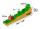A line has a rise of 2 and a run of 11. What is the slope?
• Sphere equationObtain the equation of sphere its centre on the line 3x+2z=0=4x-5y and passes through the points (0,-2,-4) and (2,-1,1).
• Vector equationLet’s v = (1, 2, 1), u = (0, -1, 3) and w = (1, 0, 7) . Solve the vector equation c1 v + c2 u + c3 w = 0 for variables c1 c2, c3 and decide weather v, u and w are linear dependent or independent
• Solve 2Solve integer equation: a +b+c =30 a, b, c = can be odd natural number from this set (1,3,5,7,9,11,13,15)
• Linear systemSolve a set of two equations of two unknowns: 1.5x+1.2y=0.6 0.8x-0.2y=2
• Perpendicular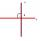Determine the slope of the line perpendicular to the line p: y = -x +4.